# Find next member

Find x if the numbers from a GP 7, 49, x.

x =  343

### Step-by-step explanation: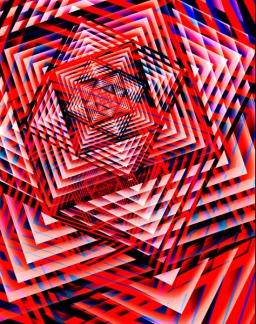Did you find an error or inaccuracy? Feel free to write us. Thank you!## Related math problems and questions:

• Gp - 80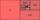One of the first four members of a geometric progression is 80. Find its if we know that the fourth member is nine times greater than the second.
• Three members GPThe sum of three numbers in GP (geometric progression) is 21 and the sum of their squares is 189. Find the numbers.
• Q of GP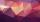Calculate quotient of geometric progression if a1=5 a a1+a2=12.
• Geometric seqFind the third member of geometric progression if a1 + a2 = 36 and a1 + a3 = 90. Calculate its quotient.
• Terms of GPWhat is the 6th term of the GP 9, 81, 729,. .. ?
• Sequences AP + GPThe three numbers that make up the arithmetic sequence have the sum of 30. If we subtract from the first 5, from the second 4 and keep the third, we get the geometric sequence. Find AP and GP members.
• GP - 8 itemsDetermine the first eight members of a geometric progression if a9=512, q=2
• Insert into GPBetween numbers 5 and 640, insert as many numbers to form geometric progression so the sum of the numbers you entered will be 630. How many numbers must you insert?
• Geometric progressionIn geometric progression, a1 = 7, q = 5. Find the condition for n to sum first n members is: sn≤217.
• Missing term 2What is the missing term for the Geometric Progression (GP) 3, 15, 75,__, 1875?
• Fifth memberDetermine the fifth member of the arithmetic progression, if the sum of the second and fifth members equal to 73, and difference d = 7.
• Progression-12, 60, -300,1500 need next 2 numbers of pattern
• GP membersThe geometric sequence has 10 members. The last two members are 2 and -1. Which member is -1/16?
• Arithmetic progression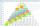In some AP applies: 5a2 + 7a5 = 90 s3 = 12 Find the first member a =? and difference d = ?
• Tenth memberCalculate the tenth member of geometric sequence when given: a1=1/2 and q=2
• Geometric progression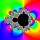Fill 4 numbers between 4 and -12500 to form geometric progression.
• Probably memberLook at the series 2,6,25,96,285, ? What number should come next?